deutsch     english    français     Print

## 11.1 FUN MIND GAMES### INTRODUCTION

 Mind games with mathematical backgrounds are very popular and wide-spread. The goal of this chapter is not to spoil your pleasure of solving such puzzles "by hand" using paper and pen. Much rather, you should experience that the computer can find the solution by solving systematically using backtracking, however, with two significant differences. On the one hand, if no other limitations and strategies are incorporated into the solution process, the solution can take an enormous amount of time, even on a fast computer. On the other hand, the computer can basically find all solutions which may be very difficult to do by hand. This makes it possible to use the computer to provide evidence that a certain solution is the simplest (shortest).

### SUDOKU

The game Sudoku has boomed and spread ever since 1980, and today it is very popular. You can find them in the puzzle section of many different daily and weekly newspapers. They work like a typical number puzzle with simple rules. In the standard version, the numbers 1 to 9 must be placed in a 9x9 grid so that every number appears exactly once in each row and each column. In addition, the grid is divided into 9 sub-grids with 3x3 cells where the numbers have to occur exactly once. At the beginning of the game, a certain number of cells are already occupied. With an ideal initial situation there should be only one solution.

Depending on the initial situation, the puzzle can be easier or more difficult to solve. An experienced Sudoku player uses certain well-known or personal strategies to solve the game. When backtracking with the computer, open cells are systematically one by one filled with numbers from 1 to 9, so that there is no conflict with the rules of the game. If there is no possibility anymore, the last turn is cancelled.

 In the following, the computer uses this backtracking algorithm. Using a GameGrid is ideal for the graphical representation, since the game has a grid structure. You draw the initial numbers in black as a TextActor, and the inserted numbers in red. As you know about backtracking from chapter 10.3. you must first find a favorable data structure for the game states. Since there is a 9x9 grid in this case, you should choose a list of 9 row lists. The number 0 indicates an empty cell. Thus, the game shown here begins with the following state: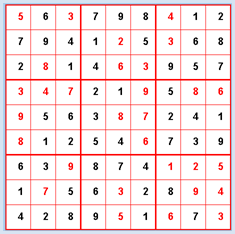startState = [ \
[0, 6, 0, 7, 9, 8, 0, 1, 2],
[7, 9, 4, 1, 0, 5, 0, 6, 8],
[2, 0, 1, 4, 0, 0, 9, 5, 7],
[0, 0, 0, 2, 1, 0, 5, 0, 0],
[0, 5, 6, 3, 0, 0, 2, 4, 1],
[0, 1, 2, 5, 4, 0, 7, 3, 9],
[6, 3, 0, 8, 7, 4, 0, 0, 0],
[1, 0, 5, 6, 0, 2, 8, 0, 0],
[4, 2, 8, 9, 0, 1, 0, 7, 0]]

As usual, you will develop the program step by step. Your first task is to display the game state in the GameGrid and give the user the ability to assign the empty cells with a mouse click. While this is unnecessary in the automatic search of the solution, it allows you to interactively influence the game, which can be especially helpful in the testing phase. Also, this way you can solve the puzzle on the screen instead of on a piece of paper.

```from gamegrid import *

def pressEvent(e):
loc = toLocationInGrid(e.getX(), e.getY())
if loc in fixedLocations:
setStatusText("Location fixed")
return
x = loc.x
y = loc.y
value = startState[y][x]
value = (value + 1)  % 10
startState[y][x] = value
showState(startState)

def showState(state):
removeAllActors()
for y in range(9):
for x in range(9):
loc = Location(x, y)
value = state[y][x]
if  value != 0:
if loc in fixedLocations:
c = Color.black
else:
c = Color.red
digit = TextActor(str(value), c, Color.white,
Font("Arial", Font.BOLD, 20))
addActorNoRefresh(digit, loc)
refresh()

makeGameGrid(9, 9, 50, Color.red, False, mousePressed = pressEvent)
show()
setTitle("Sudoku")
setBgColor(Color.white)
getBg().setLineWidth(3)
getBg().setPaintColor(Color.red)
for x in range(4):
getBg().drawLine(150 * x, 0, 150 * x, 450)
for y in range(4):
getBg().drawLine(0, 150 * y, 450, 150 * y)

startState = [
[0, 6, 0, 7, 9, 8, 0, 1, 2],
[7, 9, 4, 1, 0, 5, 0, 6, 8],
[2, 0, 1, 4, 0, 0, 9, 5, 7],
[0, 0, 0, 2, 1, 0, 5, 0, 0],
[0, 5, 6, 3, 0, 0, 2, 4, 1],
[0, 1, 2, 5, 4, 0, 7, 3, 9],
[6, 3, 0, 8, 7, 4, 0, 0, 0],
[1, 0, 5, 6, 0, 2, 8, 0, 0],
[4, 2, 8, 9, 0, 1, 0, 7, 0]]

fixedLocations = []
for x in range(9):
for y in range(9):
if startState[y][x] != 0:
fixedLocations.append(Location(x, y))

showState(startState)
```

Highlight program code (Ctrl+C copy, Ctrl+V paste)

 The next step is to build a function isValid(state) that checks if a given game state state complies with the rules of the game. This is tedious work because you have to check the 9 rows and 9 columns, as well as the 9 square blocks. You can write out the result to the status bar.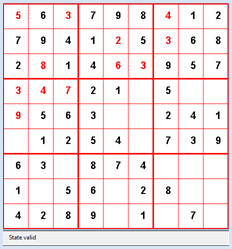```from gamegrid import *

def pressEvent(e):
loc = toLocationInGrid(e.getX(), e.getY())
if loc in fixedLocations:
setStatusText("Location fixed")
return
xs = loc.x // 3
ys = loc.y // 3
x = loc.x % 3
y = loc.y % 3
value = startState[ys][xs][y][x]
value = (value + 1)  % 10
startState[ys][xs][y][x] = value
showState(startState)
if isValid(startState):
setStatusText("State valid")
else:
setStatusText("Invalid state")

def showState(state):
removeAllActors()
for ys in range(3):
for xs in range(3):
for y in range(3):
for x in range(3):
loc = Location(x + 3 * xs, y + 3 * ys)
value = state[ys][xs][y][x]
if  value != 0:
if loc in fixedLocations:
c = Color.black
else:
c = Color.red
digit = TextActor(str(value), c, Color.white,
Font("Arial", Font.BOLD, 20))
addActorNoRefresh(digit, loc)
refresh()

def isValid(state):
# Check lines
for ys in range(3):
for y in range(3):
line = []
for xs in range(3):
for x in range(3):
value = state[ys][xs][y][x]
if value > 0 and value in line:
return False
else:
line.append(value)
# Check rows
for xs in range(3):
for x in range(3):
row = []
for ys in range(3):
for y in range(3):
value = state[ys][xs][y][x]
if value > 0 and value in row:
return False
else:
row.append(value)

# Check subgrids
for ys in range(3):
for xs in range(3):
subgrid = state[ys][xs]
square = []
for y in range(3):
for x in range(3):
value = subgrid[y][x]
if value > 0 and value in square:
return False
else:
square.append(value)
return True

makeGameGrid(9, 9, 50, Color.red, False, mousePressed = pressEvent)
show()
setTitle("Sudoku")
addStatusBar(30)
visited = []
setBgColor(Color.white)
getBg().setLineWidth(3)
getBg().setPaintColor(Color.red)
for x in range(4):
getBg().drawLine(150 * x, 0, 150 * x, 450)
for y in range(4):
getBg().drawLine(0, 150 * y, 450, 150 * y)

stateWiki = [[[[0, 3, 0], [0, 0, 0], [0, 0, 8]],
[[0, 0, 0], [1, 9, 5], [0, 0, 0]],
[[0, 0, 0], [0, 0, 0], [0, 6, 0]]],
[[[8, 0, 0], [4, 0, 0], [0, 0, 0]],
[[0, 6, 0], [8, 0, 0], [0, 2, 0]],
[[0, 0, 0], [0, 0, 1], [0, 0, 0]]],
[[[0, 6, 0], [0, 0, 0], [0, 0, 0]],
[[0, 0, 0], [4, 1, 9], [0, 0, 0]],
[[2, 8, 0], [0, 0, 5], [0, 7, 0]]]]
startState = stateWiki

fixedLocations = []
for xs in range(3):
for ys in range(3):
for x in range(3):
for y in range(3):
if startState[ys][xs][y][x] != 0:
fixedLocations.append(Location(x + 3 * xs, y + 3 * ys))

showState(startState)
```

Highlight program code (Ctrl+C copy, Ctrl+V paste)

For the backtracking, you have to determine the subsequent nodes of a state with the function getNeighbours(state). For this, you choose an empty cell with getEmptyCell() and insert all numbers in order which belong to a legal game state. At least one of them will be the correct one.

In getNeighbours(state), you first copy the given state list into a clone, since you are not allowed to overwrite the state received as a parameter. Then, you fill the empty cells successively with the numbers 1 to 9 and add copies of the allowed states into the neighbor list, which you then finally return [more... A clone is created which the function deepCopy() from module copy].

You can adopt the recursively defined function search(), which performs the actual backtracking, almost without changing anything from other backtracking problems. In this case, you are looking for just a single solution and you end the recursive call with the flag found.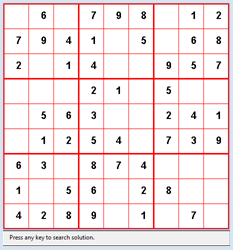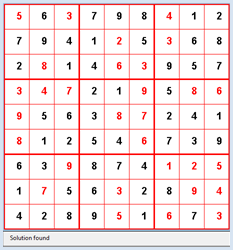```from gamegrid import *

def pressEvent(e):
loc = toLocationInGrid(e.getX(), e.getY())
if loc in fixedLocations:
setStatusText("Location fixed")
return
x = loc.x
y = loc.y
value = startState[y][x]
value = (value + 1)  % 10
startState[y][x] = value
showState(startState)
if isValid(startState):
setStatusText("State valid")
else:
setStatusText("Invalid state")

def getBlockValues(state, x, y):
return [state[y][x], state[y][x + 1], state[y][x + 2],
state[y + 1][x], state[y + 1][x + 1], state[y + 1][x + 2],
state[y + 2][x], state[y + 2][x + 1], state[ y + 2][x + 2]]

def showState(state):
removeAllActors()
for y in range(9):
for x in range(9):
loc = Location(x, y)
value = state[y][x]
if  value != 0:
if loc in fixedLocations:
c = Color.black
else:
c = Color.red
digit = TextActor(str(value), c, Color.white,
Font("Arial", Font.BOLD, 20))
addActorNoRefresh(digit, loc)
refresh()

def isValid(state):
# Check lines
for y in range(9):
values = []
for x in range(9):
value = state[y][x]
if value > 0 and value in values:
return False
else:
values.append(value)
# Check rows
for x in range(9):
values = []
for y in range(9):
value = state[y][x]
if value > 0 and value in values:
return False
else:
values.append(value)

# Check blocks
for yblock in range(3):
for xblock in range(3):
values = []
li = getBlockValues(state, 3 * xblock, 3 * yblock)
for value in li:
if value > 0 and value in values:
return False
else:
values.append(value)
return True

def getEmptyCell(state):
emptyCells = []
for y in range(9):
for x in range(9):
if state[y][x] == 0:
return [x, y]
return []

def cloneState(state):
li = []
for y in range(9):
line = []
for x in range(9):
line.append(state[y][x])
li.append(line)
return li

def getNeighbours(state):
clone = cloneState(state)
cell = getEmptyCell(state)
validStates = []
for value in range(1, 10):
clone[cell][cell] = value
if isValid(clone):
validStates.append(cloneState(clone))
return validStates

def search(state):
global found, solution
if found:
return
visited.append(state)  # state marked as visited

# Check for solution
if getEmptyCell(state) == []:
solution = state
found = True
return

for neighbour in getNeighbours(state):
if neighbour not in visited: # Check if already visited
search(neighbour) # recursive call
visited.pop()

makeGameGrid(9, 9, 50, Color.red, False, mousePressed = pressEvent)
show()
setTitle("Sudoku")
addStatusBar(30)
visited = []
setBgColor(Color.white)
getBg().setLineWidth(3)
getBg().setPaintColor(Color.red)
for x in range(4):
getBg().drawLine(150 * x, 0, 150 * x, 450)
for y in range(4):
getBg().drawLine(0, 150 * y, 450, 150 * y)

startState = [
[0, 6, 0, 7, 9, 8, 0, 1, 2],
[7, 9, 4, 1, 0, 5, 0, 6, 8],
[2, 0, 1, 4, 0, 0, 9, 5, 7],
[0, 0, 0, 2, 1, 0, 5, 0, 0],
[0, 5, 6, 3, 0, 0, 2, 4, 1],
[0, 1, 2, 5, 4, 0, 7, 3, 9],
[6, 3, 0, 8, 7, 4, 0, 0, 0],
[1, 0, 5, 6, 0, 2, 8, 0, 0],
[4, 2, 8, 9, 0, 1, 0, 7, 0]]

fixedLocations = []
for x in range(9):
for y in range(9):
if startState[y][x] != 0:
fixedLocations.append(Location(x, y))

showState(startState)
setStatusText("Press any key to search solution.")
getKeyCodeWait(True)
setStatusText("Searching. Please wait...")
found = False
solution = None
search(startState)
if solution != None:
showState(solution)
setStatusText("Solution found")
else:
setStatusText("No solution")
```

Highlight program code (Ctrl+C copy, Ctrl+V paste)

### MEMO

 You can also use Sudokus that you find on the Internet or in a puzzle section. If you have enough patience, your program should always be able to find a solution. You can greatly reduce the computation time if you include the solution strategies that you would also use when solving by hand. It is up to you to improve the algorithm with such heuristic processes. The process that you use to solve the Sudoku can also be applied to Sudoku puzzles that have non-square cells. For this, you only need to adjust the function getBlockValues() accordingly.

### THE JEALOUS HUSBANDS

 Already in 1613 the mathematician C. G. Bachet, Sieur de Méziriac published a study entitled Problèmes plaisants et détectables qui se font par les nombres, in which he describes the puzzle Les vilains maris jaloux described by the following in both French and English: "Trois maris jaloux se trouvent le soir avec leurs femmes au passage d'une rivière, et rencontrent un bateau sans batelier; le bateau est si petit, qu'il ne peut porter plus de deux personnes à la fois. On demande comment ces six personnes passeront de tel sorte qu'aucune femme ne demeure en la compagnie d'un ou de deux hommes, si son mari n'est présent, soit sur l'une des deux rives, soit sur le bateau." (Lit. Édouard Lucas, L'arithmétique amusante" 1885, reprint 2006)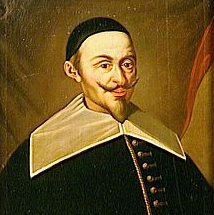Claude Gaspard Bachet de Méziriac (1581-1638) (© Wiki)

"Three jealous husbands find themselves with their wives at a river crossing in the evening where they find a boat without a boatman. The boat is so small that it only fits two people. This poses the question of how all six people can cross the river without ever putting one of the women in the presence of one or two men without her own husband being present, on either side of the land or in the boat."

You again solve this problem step by step. First you create the user interface (a GameGrid works well for this) since the people can be easily represented by sprite images. Add the GameGrid actors to an invisible 7x3 grid so that you can draw a river on the middle strip. Since it is only really important if a person is to the left or the right of the river, a binary number is suitable as a data structure where each bit belongs to a particular person. The bit value 0 means that the person is on the left side of the river, and the value 1 means that they are on the right side. Use the bit with the highest value for the boat.

In the simulation program there is a blue, a green, and a red couple that you related to the digits of the binary number as follows:

 b6 b5 b4 b3 b2 b1 b0 boat man_red female_red man_green female_green man_blue female_blue

b0 is the bit with the smallest value. If you interpret the state in the decimal system, all numbers between 0 and 127 occur, i.e. there are 128 different states.

 It is highly recommended that you take a small detour here so that you can try the coding of the states in a test program. The image jumps from one side of the river to the other when you click on a person or on the boat. The state is written out to the title bar in both its decimal and its binary notation.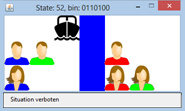You already build a test here with isStateAllowed(state) to check if the current situation is legal according to the rules. (You could also first create a prototype without this test.)

```from gamegrid import *

def pressEvent(e):
global state
loc = toLocationInGrid(e.getX(), e.getY())
if loc in left_locations:
actor = getOneActorAt(loc)
if actor != None:
x = 6 - actor.getX()
y = actor.getY()
actor.setLocation(Location(x, y))
if loc in right_locations:
actor = getOneActorAt(loc)
if actor != None:
x = 6 - actor.getX()
y = actor.getY()
actor.setLocation(Location(x, y))
state = 0
for i in range(7):
loc = right_locations[i]
actor = getOneActorAt(loc)
if actor != None:
state += 2**(6 - i)
showState(state)

def stateToString(state):
return str(bin(state)[2:]).zfill(7)

def showState(state):
sbin = stateToString(state)
for i in range(7):
if sbin[i] == "0":
actors[i].setLocation(left_locations[i])
else:
actors[i].setLocation(right_locations[i])
setTitle("State: " + str(state) + ", bin: " + stateToString(state))
if isStateAllowed(state):
setStatusText("Situation allowed")
else:
setStatusText("Situation not allowed")
refresh()

def isStateAllowed(state):
print state
stateStr = stateToString(state)
mred = stateStr == "1"
fred = stateStr == "1"
mgreen = stateStr == "1"
fgreen = stateStr == "1"
mblue = stateStr == "1"
fblue = stateStr == "1"

if mred and not fred or not mred and fred: # mred/fred not together
if not fred and (not mgreen or not mblue) or fred and (mgreen or mblue):
return False
if mgreen and not fgreen or not mgreen and fgreen:#mgreen/fgreen not together
if not fgreen and (not mred or not mblue) or fgreen and (mred or mblue):
return False
if mblue and not fblue or not mblue and fblue:  # mblue/fblue not together
if not fblue and (not mred or not mgreen) or fblue and (mred or mgreen):
return False
return True

makeGameGrid(7, 3, 50, None, False, mousePressed = pressEvent)
setBgColor(Color.white)
addStatusBar(30)
show()
actors = [Actor("sprites/boat.png"),
Actor("sprites/man_0.png"), Actor("sprites/woman_0.png"),
Actor("sprites/man_1.png"), Actor("sprites/woman_1.png"),
Actor("sprites/man_2.png"), Actor("sprites/woman_2.png")]

left_locations =  [Location(2, 0),
Location(2, 1), Location(2, 2),
Location(1, 1), Location(1, 2),
Location(0, 1), Location(0, 2)]
right_locations = [Location(4, 0),
Location(4, 1), Location(4, 2),
Location(5, 1), Location(5, 2),
Location(6, 1), Location(6, 2)]

for i in range(7):
addActorNoRefresh(actors[i], left_locations[i])
for i in range(3):
getBg().fillCell(Location(3, i), Color.blue)
refresh()

startState = 0
showState(startState)
```

Highlight program code (Ctrl+C copy, Ctrl+V paste)

In the next step you implement the backtracking algorithm, as you know it from chapter 10.3. Again, you first have to determine all possible successive states for a given state in getNeighbours(state). You begin as follows: First you distinguish whether the boat is located on the left side of the river (state < 64) or the right (state >=64). Then you determine all people who are available to move across the river either as an individual or in a two person team from the lists li_one and li_two. When using removeForbiddenTransfers() you also must consider that a woman can never be in the boat with a man that is not her husband.

You implement the backtracking in its familiar form in search(). You copy the solutions into a list named solutions, so that you can access them at the end of the search process to examine the solutions. Thereby you first write out the number of found solutions and then select the shortest one which can then be run through using key press.

```from gamegrid import *
import itertools

def pressEvent(e):
global state
loc = toLocationInGrid(e.getX(), e.getY())
if loc in left_locations:
actor = getOneActorAt(loc)
if actor != None:
x = 6 - actor.getX()
y = actor.getY()
actor.setLocation(Location(x, y))
if loc in right_locations:
actor = getOneActorAt(loc)
if actor != None:
x = 6 - actor.getX()
y = actor.getY()
actor.setLocation(Location(x, y))
state = 0
for i in range(7):
loc = right_locations[i]
actor = getOneActorAt(loc)
if actor != None:
state += 2**(6 - i)
setTitle("State: " + str(state) + ", bin: " + stateToString(state))
if isStateAllowed(state):
setStatusText("Situation allowed")
else:
setStatusText("Situation not allowed")
refresh()

def stateToString(state):
return str(bin(state)[2:]).zfill(7)

def showState(state):
sbin = stateToString(state)
for i in range(7):
if sbin[i] == "0":
actors[i].setLocation(left_locations[i])
else:
actors[i].setLocation(right_locations[i])
refresh()

def getTransferInfo(state1, state2):
state1 = state1 & 63
state2 = state2 & 63
mod = state1 ^ state2
passList = []
for n in range(6):
if mod % 2 == 1:
if n // 2 == 0:
couple = "blue"
elif n // 2 == 1:
couple = "green"
elif n // 2 == 2:
couple = "red"
if n % 2 == 0:
passList.append("f" + couple)
else:
passList.append("m" + couple)
mod = mod // 2
return passList

def getTransferSequence(solution):
transferSequence = []
oldState = solution
for state in solution[1:]:
transferSequence.append(getTransferInfo(oldState, state))
oldState = state
return transferSequence

def isStateAllowed(state):
stateStr = stateToString(state)
mred = stateStr == "1"
fred = stateStr == "1"
mgreen = stateStr == "1"
fgreen = stateStr == "1"
mblue = stateStr == "1"
fblue = stateStr == "1"

if mred and not fred or not mred and fred:  # mred/fred not together
if not fred and (not mgreen or not mblue) or fred and (mgreen or mblue):
return False
if mgreen and not fgreen or not mgreen and fgreen:#mgreen/fgreen not together
if not fgreen and (not mred or not mblue) or fgreen and (mred or mblue):
return False
if mblue and not fblue or not mblue and fblue:  # mblue/fblue not together
if not fblue and (not mred or not mgreen) or fblue and (mred or mgreen):
return False
return True

def removeForbiddenTransfers(li):
forbiddenPairs = [(0, 3), (0, 5), (1, 2), (2, 5), (1, 4), (3, 4)]
allowedPairs = []
for pair in li:
if pair not in forbiddenPairs:
allowedPairs.append(pair)
return allowedPairs

def getNeighbours(state):
neighbours = []
li_one = []  # one person in boat
bin = stateToString(state)
if state < 64:  # boat at left
for i in range(6):
if bin[6 - i] == "0":
li_one.append(i)
li_two = list(itertools.combinations(li_one, 2)) #two persons in boat
li_two = removeForbiddenTransfers(li_two)
else: # boat at right
for i in range(6):
if bin[6 - i] == "1":
li_one.append(i)
li_two = list(itertools.combinations(li_one, 2))
li_two = removeForbiddenTransfers(li_two)

li_values = []
if state < 64:  # boat at left, restrict to two persons transfer
for li in li_two:
li_values.append(2**li + 2**li + 64)
else:  # boat at right, one or two persons transfer
for i in li_one:
li_values.append(2**i + 64)
for li in li_two:
li_values.append(2**li + 2**li + 64)

for value in li_values:
v = state ^ value
if isStateAllowed(v):  # restrict to allowed states
neighbours.append(v)
return neighbours

def search(state):
visited.append(state)  # state marked as visited

# Check for solution
if state == targetState:
solutions.append(visited[:])

for neighbour in getNeighbours(state):
if neighbour not in visited: # Check if already visited
search(neighbour) # recursive call
visited.pop()

nbSolution = 0
makeGameGrid(7, 3, 50, None, False, mousePressed = pressEvent)
addStatusBar(30)
setBgColor(Color.white)
setTitle("Searching...")
show()
visited = []
actors = [Actor("sprites/boat.png"),
Actor("sprites/man_0.png"), Actor("sprites/woman_0.png"),
Actor("sprites/man_1.png"), Actor("sprites/woman_1.png"),
Actor("sprites/man_2.png"), Actor("sprites/woman_2.png")]

left_locations =  [Location(2, 0),
Location(2, 1), Location(2, 2),
Location(1, 1), Location(1, 2),
Location(0, 1), Location(0, 2)]
right_locations = [Location(4, 0),
Location(4, 1), Location(4, 2),
Location(5, 1), Location(5, 2),
Location(6, 1), Location(6, 2)]

for i in range(7):
addActorNoRefresh(actors[i], left_locations[i])
for i in range(3):
getBg().fillCell(Location(3, i), Color.blue)
refresh()

startState = 0
targetState = 127
solutions = []
search(startState)

maxLength = 0
maxSolution = None
minLength = 100
minSolution = None
for solution in solutions:
if len(solution) > maxLength:
maxLength = len(solution)
maxSolution = solution
if len(solution) < minLength:
minLength = len(solution)
minSolution = solution
setStatusText("#Solutions: " + str(len(solutions)) + ", Min Length: "
+ str(minLength) + ", Max Length: " + str(maxLength))

setTitle("Press key to cycle")
oldState = startState
for state in minSolution[1:]:
getKeyCodeWait(True)
showState(state)
info = getTransferInfo(oldState, state)
setTitle("#Transferred: " + str(info))
oldState = state
setTitle("Done. #Boat Transfers: " + str((len(minSolution) - 1)))
```

Highlight program code (Ctrl+C copy, Ctrl+V paste)

### MEMO

 Puzzles of this type have the feature that you do not know whether they have exactly one or multiple solutions from the start. It turns out that it is much easier for us humans to find a solution, than it is to prove that there is no solution. For the computer which systematically searches for all solutions with an exhaustive search, the search for all solutions is generally not a problem except that it can take a long time. This is unfortunately already the case with relatively simple games, due to combinatorial explosion, so we again bump into the limits of using the computer. It is interesting that Bachet had already published the solution with minimum amount of 11 crossings, which is also what your computer program finds. He argued so skillfully crossing by crossing that the strategy of solving the puzzle appears to be evident. However, whether he found the solution first by “trial and error”, and only later wrote the arguments for it, remains undecided.

### EXERCISES

 1 Find a solution for the following X-Sudoku, in which the 9 numbers can also only appear once on either of the two main diagonals.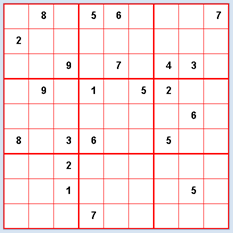2 Show that there is no solution to the problem of "The Jealous Husbands" if only a single person is allowed to cross the river from right to left at a time.